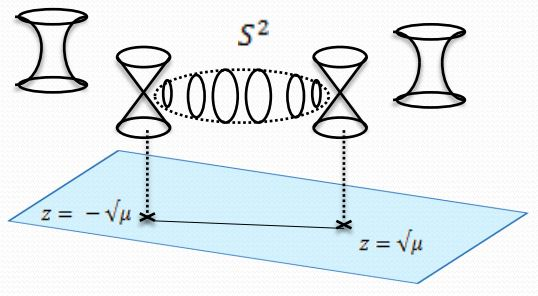Contents

# Contents

## Idea

A nodal singularity of an algebraic curve is one of the form parameterized by the equation $x y = 0$. A nodal curve is a curve with a nodal singularity.

(e.g. Hain 08, p. 45)

## The nodal cubic

The nodal cubic curve (over some base) is (see at elliptic curve – Nodal curves and cuspidal curves for notation and background) the solution to the Weierstrass equation for which the discriminant vanishes, but the modular invariant $c_4$ does not.

This is equivalently the limit in which the j-invariant $j = \frac{c_4^3}{\Delta}$ goes to $\infty$.

## Properties of the nodal cubic

### Compactified moduli stack of elliptic curves and the Tate curve

The nodal cubic curve is not an elliptic curve, as it is singular, but adding it to the moduli stack of elliptic curves $\mathcal{M}_{ell}$ produces the compactification $\mathcal{M}_{\overline{ell}}$ which is often relevant.

The formal neighbourhood of the nodal curve in $\mathcal{M}_{\overline{ell}}$ is the Tate curve.

### Over the complex numbers

Over the complex numbers, the nodal cubic $E_0$ is the Riemann sphere/complex projective space $\mathbb{P}^1$ with the pole points 0 and $\infty$ identified (hence is a “complex torus with one cycle shrunk away”). Precisely: there is a holomorphic function

$\mathcal{P}^1 \to \mathcal{P}^2$

which is onto $E_0 \subset \mathcal{P}_2$, sends the unit of the multiplicative group $1 \in \mathbb{C}^\times \hookrightarrow \mathbb{P}^1$ to the unit of $E_0$, maps $0,\infty \in \mathbb{P}^1$ both to the nodal singular double point of $E_0$ and is injective away from these points (e.g. Hain 08, exercise 47, p. 45)

$\array{ \mathbb{C}^\times &\hookrightarrow& E_0 \\ \downarrow^\mathrlap{=} && \downarrow \\ \mathbb{P}^1-\{0,\infty\} &\longrightarrow& \mathbb{P}^2 } \,.$### Formal group and height

The formal group associated with a nodal cubic curve is of height 1. Indeed, passing to the point of the nodal curve in $\mathcal{M}_{\overline{ell}}$ connects elliptic cohomology (of chromatic level 2) to topological K-theory (of chromatic level 1). For more on this see at moduli stack of tori and at tmf – Properties – Maps to K-theory and to Tate K-theory.

### Relation to gauge enhancement in F-theory

In F-theory the nodal singularity locus of the given elliptic fibration is interpreted as the locus of D7-branes, see at F-brane scan.

Discussion over the complex numbers is in